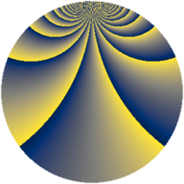# Properties

 Label 475.3.bjLevel $475$ Weight $3$ Character orbit 475.bj Rep. character $\chi_{475}(17,\cdot)$ Character field $\Q(\zeta_{180})$ Dimension $4704$ Sturm bound $150$

# Related objects

## Defining parameters

 Level: $$N$$ $$=$$ $$475 = 5^{2} \cdot 19$$ Weight: $$k$$ $$=$$ $$3$$ Character orbit: $$[\chi]$$ $$=$$ 475.bj (of order $$180$$ and degree $$48$$) Character conductor: $$\operatorname{cond}(\chi)$$ $$=$$ $$475$$ Character field: $$\Q(\zeta_{180})$$ Sturm bound: $$150$$

## Dimensions

The following table gives the dimensions of various subspaces of $$M_{3}(475, [\chi])$$.

Total New Old
Modular forms 4896 4896 0
Cusp forms 4704 4704 0
Eisenstein series 192 192 0

## Trace form

 $$4704q - 48q^{2} - 48q^{3} - 60q^{4} - 48q^{5} - 36q^{6} - 42q^{7} - 24q^{8} - 60q^{9} + O(q^{10})$$ $$4704q - 48q^{2} - 48q^{3} - 60q^{4} - 48q^{5} - 36q^{6} - 42q^{7} - 24q^{8} - 60q^{9} - 48q^{10} - 18q^{11} - 24q^{12} - 48q^{13} - 60q^{14} - 66q^{15} - 36q^{16} - 102q^{17} - 288q^{18} - 60q^{19} - 156q^{20} - 36q^{21} - 264q^{22} + 48q^{23} + 48q^{25} - 48q^{26} - 24q^{27} + 372q^{28} + 240q^{29} - 24q^{30} - 18q^{31} + 330q^{32} + 618q^{33} - 60q^{34} + 114q^{35} - 36q^{36} - 96q^{37} - 924q^{38} - 120q^{39} - 1344q^{40} - 36q^{41} - 264q^{42} + 1236q^{43} - 60q^{44} + 174q^{45} - 18q^{46} - 528q^{47} - 1092q^{48} + 264q^{50} - 96q^{51} + 48q^{52} - 30q^{53} - 60q^{54} + 42q^{55} - 72q^{56} + 1986q^{57} + 48q^{58} + 1140q^{59} - 912q^{60} - 36q^{61} - 3384q^{62} - 42q^{63} - 30q^{64} + 1056q^{65} - 468q^{66} + 648q^{67} - 360q^{68} - 30q^{69} - 720q^{70} - 36q^{71} - 204q^{72} - 210q^{73} + 576q^{75} - 96q^{76} - 1128q^{77} - 1008q^{78} - 60q^{79} + 708q^{80} - 36q^{81} - 2064q^{82} - 1758q^{83} - 3630q^{84} + 576q^{85} - 36q^{86} + 1944q^{87} - 1152q^{88} - 60q^{89} - 3096q^{90} - 36q^{91} - 2100q^{92} + 90q^{93} - 120q^{94} - 276q^{95} - 72q^{96} - 252q^{97} + 966q^{98} + O(q^{100})$$

## Decomposition of $$S_{3}^{\mathrm{new}}(475, [\chi])$$ into newform subspaces

The newforms in this space have not yet been added to the LMFDB.